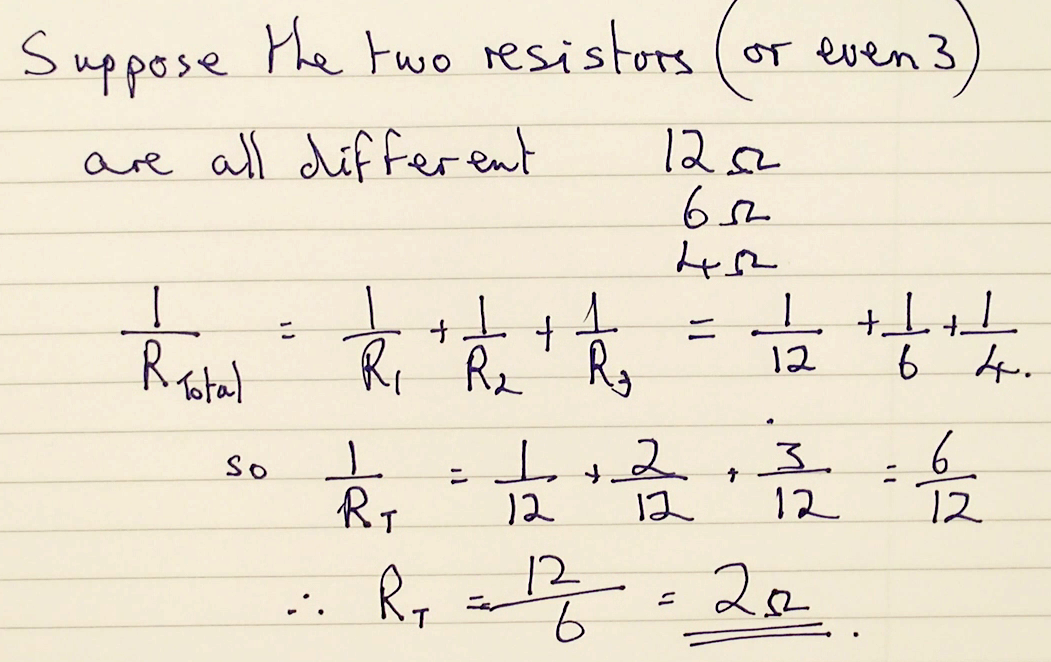### Resistors in parallel

When resistors are connected in parallel the current has more than one path that it can take, therefore a larger current will flow. Because of this is the total resistance is always going to be less than either of the individual resistors.

In the photograph below two resistors are connected in parallel and have a current flowing through. The total current is being measured as is the potential difference across both the resistors. From these two values therefore, we can calculate the total resistance.

Using the equation that R the resistance equals potential difference divided by current we calculate the total resistance:And you can see that the total resistance is very much less than any of the individual values